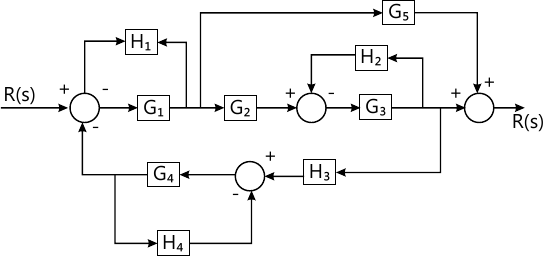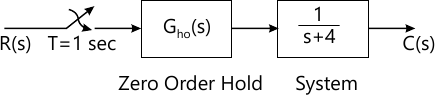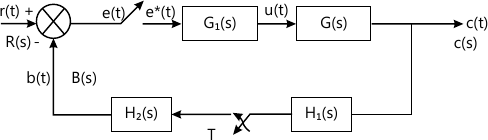Total marks: --
Total time: --
INSTRUCTIONS
(1) Assume appropriate data and state your reasons
(2) Marks are given to the right of every question
(3) Draw neat diagrams wherever necessary

Answer any one question from Q1 and Q2
1 (a) Explain the rules of block diagram reduction techniques.
6 M
1 (b) f peak overshoot is 16.3% and peak time is 0.3023 seconds. Determine:
(1) damping factor,
(2) undamped natural frequency and
6 M

2 (a) Find the closed loop transfer function $\dfrac {C(s)} {R(s)}$ of system shown in Fig. 1 using block diagram reduction technique.6 M
2 (b) $If G(s) \ H(s) = \dfrac {25}{s(s+5)},$ obtain damping factor, un-damped and damped natural frequency, rise time, peak time, and settling time.
6 M

Answer any one question from Q3 and Q4
3 (a) Comment on the stability of a system using Routh's stability criteria whose characteristic equation is:
s4+2s3+4s2+6s+8=0.
How many poles of systems lie in right half of s-plane?
4 M
3 (b) $If G(s) \ H(s) = \dfrac {24} {s(s+2)(s+12)},$ construct the Bode plot and calculate gain crossover frequency, phase crossover frequency, gain margin, phase margin and comment on stability.
8 M

4 (a) Open loop transfer function of unity feedback system is $G(s) = \dfrac {K}{s(s+3)(s+5)}$ Sketch the complete root locus and find marginal gain.
8 M
4 (b) $If G(s) \ H(s) = \dfrac {1} {s(s+1)},$ determine the value of:
i) Resonance Peak and
ii) Resonance frequency.
4 M

Answer any one question from Q5 and Q6
5 (a) State any three advantages of state space approach over classical approach. Derive an expression to obtain transfer function from state model.
7 M
5 (b) Find Controllability and Observability of the system given by state model: $A= \begin{bmatrix} 1 &1 &5 \\1 &-2 &2 \\5 &2 &-8 \end{bmatrix}, \ B=\begin{bmatrix} 5 \\ 1\\10 \end{bmatrix}, \ C=\begin{bmatrix} 10 &15 &11 \end{bmatrix}, \ D=$
6 M

6 (a) Explain canonical controllable and observable state model with any example/transfer function.
6 M
6 (b) Obtain the state transition matrix for the system with state equation: $[x]= \begin{bmatrix} 0 &1 \\-8 &-9 \end{bmatrix}$ using Laplace transformation.
7 M

Answer any one question from Q7 and Q8
7 (a) Explain application of programmable logic controller for elevator system with ladder diagram.
6 M
7 (b) Find the pulse transfer function and impulse response of the system shown in Fig. 2.7 M

8 (a) Write the equation of PID controller and explain role of each action in short.
6 M
8 (b) Obtain pulse transfer function of the system shown in Fig. 3 using first (Starred Laplace) principle.7 M

More question papers from Control Systems